Courses

# Radiation - MCQ Test 2

## 30 Questions MCQ Test GATE Mechanical (ME) 2022 Mock Test Series | Radiation - MCQ Test 2

Description
This mock test of Radiation - MCQ Test 2 for Mechanical Engineering helps you for every Mechanical Engineering entrance exam. This contains 30 Multiple Choice Questions for Mechanical Engineering Radiation - MCQ Test 2 (mcq) to study with solutions a complete question bank. The solved questions answers in this Radiation - MCQ Test 2 quiz give you a good mix of easy questions and tough questions. Mechanical Engineering students definitely take this Radiation - MCQ Test 2 exercise for a better result in the exam. You can find other Radiation - MCQ Test 2 extra questions, long questions & short questions for Mechanical Engineering on EduRev as well by searching above.
QUESTION: 1

Solution:

Ans. (d)

QUESTION: 2

Solution:

Ans. (d)

QUESTION: 3

### Assertion (A): In a furnace, radiation from the walls has the samewavelength as the incident radiation from the heat source. Reason (R): Surfaces at the same temperature radiate at the samewavelength.

Solution:

Ans. (d) Wall and furnace has different temperature.

QUESTION: 4

Consider the following statements:
1. For metals, the value of absorptivity is high
.2. For non-conducting materials, reflectivity is low.
3. For polished surfaces, reflectivity is high.
4. For gases, reflectivity is very low.Of these statements:

Solution:

Ans. (c)

QUESTION: 5

Assertion (A): Heat transfer at high temperature is dominated byradiation rather than convection.

Reason (R): Radiation depends on fourth power of temperature while convection depends on unit power relationship.

Solution:

Ans. (a)

QUESTION: 6

Consider following parameters:
1. Temperature of the surface
2. Emissivity of the surface
3. Temperature of the air in the room
4. Length and diameter of the pipe
The parameter(s) responsible for loss of heat from a hot pipe surface ina room without fans would include

Solution:

Ans. (d) All parameters are responsible for loss of heat from a hot pipe surface.

QUESTION: 7

Match List-I (Type of radiation) with List-II (Characteristic) and select the correct answer: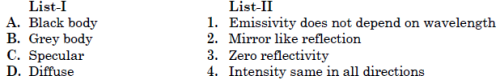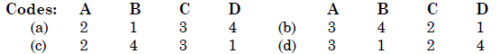Solution:

Ans. (d)

QUESTION: 8

Assertion (A): Solar Radiation is mainly scattered or transmitted butnot absorbed by the atmosphere. Reason (R): Absorptivity of atmosphere is low.

Solution:

Ans. (a)

QUESTION: 9

A body at 500 K cools by radiating heat to ambient atmosphere maintained at 300 K. When the body has cooled to 400 K, the coolingrate as a percentage of original cooling rate is about.

Solution:

Ans. (a)

QUESTION: 10

What is the ratio of thermal conductivity to electrical conductivity equal to?

Solution:

Ans. (c)

QUESTION: 11

A pipe carrying saturated steam is covered with a layer of insulationand exposed to ambient air.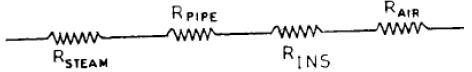The thermal resistances are as shown in the figure.Which one of the following statements is correct in this regard?

Solution:

Ans. (a) The resistance due to steam film and pipe material are negligible in comparison to resistance of insulation material and resistance due to air film.

QUESTION: 12

Using thermal-electrical analogy in heat transfer, match List-I (Electrical quantities) with List-II (Thermal quantities) and select the correct answer: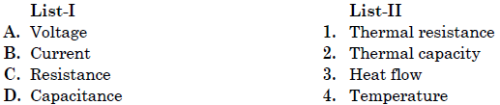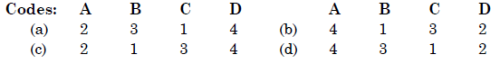Solution:

Ans. (d)

QUESTION: 13

What is the equivalent emissivity for radiant heat exchange between asmall body (emissivity = 0.4) in a very large enclosure (emissivity = 0·5)?

Solution:

Ans. (b)

QUESTION: 14

With reference to the abovefigure, the shape factorbetween 1 and 2 is: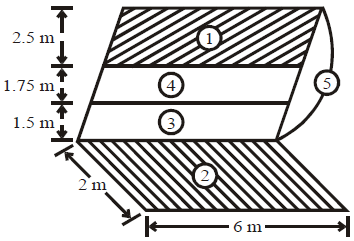Solution:

Ans. (d)

QUESTION: 15

Three black bodies A, B and C in the form of cubes of sides in the ratio of 3: 4: 5 are kept at the same high temperature. The ratio of the quantity of heat lost per second by A, B and C will be

Solution:
QUESTION: 16

The earth receives at its surface radiation from the sun at the rate of 1400 W/m2. The distance of centre of sun from the surface of earth is1.5 × 108 m and the radius of sun is 7.0 × 108 m. What is approximately the surface temperature of the sun treating the sun as a black body?

Solution:

Ans. (c)

QUESTION: 17

Which one of the following statements is correct? For a hemisphere, the solid angle is measured.

Solution:

Ans. (c)

QUESTION: 18

The wave length of the radiation emitted by a body depends upon

Solution:

Ans. (c)

QUESTION: 19

What is the basic equation of thermal radiation from which all other equations of radiation can be derived?

Solution:

Ans. (b)

QUESTION: 20

Two spheres A and B of same material have radii 1 m and 4 m and temperature 4000 K and 2000 K respectively. Which one of the following statements is correct? The energy radiated by sphere A is:

Solution:

Ans. (c)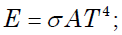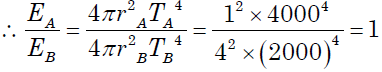QUESTION: 21

If the temperature of a solid state changes from 27°C to 627°C, the nemissive power changes which rate

Solution:

Ans. (d)
Emissivepower (E) =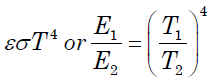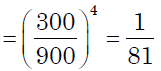QUESTION: 22

A hollow encloser is formed betweentwo infinitely long concentric cylindersof radii 1 m ans 2 m, respectively.Radiative heat exchange takes placebetween the inner surface of the larger cylinder (surface-2) and the outersurface of the smaller cylinder (surface-I). The radiating surfaces are diffuseand the medium in the enclosure isnon-participating. The fraction of thethermal radiation leaving the largersurface and striking itself is :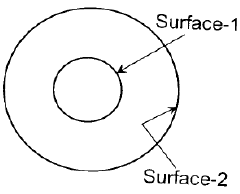Solution:

Ans. (b) It is shape factor =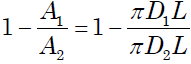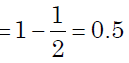QUESTION: 23

What is the value of the view factor for two inclined flat plates having common edge of equal width, and with an angle of 20 degrees?

Solution:

Ans. (a)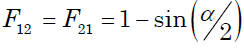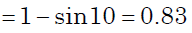QUESTION: 24

A spherical aluminium shell of inside diameter 2 m is evacuated andused as a radiation test chamber. If the inner surface is coated with carbon black and maintained at 600 K, the irradiation on a small test surface placed inside the chamber is:
(Stefan-Boltzmann constant σ = 5.67 × 10-8 W/m2K4)

Solution:

Ans. (d) Irradiation on a small test surface placed inside a hollow black spherical chamber = σT4 = 5.67 × 10-8 × 6004 = 7348 W/m2

QUESTION: 25

A large spherical enclosure has a small opening. The rate of emission of radiative flux through this opening is 7.35 kW/m2. The temperature atthe inner surface of the sphere will be about (assume Stefan Boltzmann constants σ = 5.67 × 10-8 W/m2K4)

Solution:

Ans. (a) Rate of emission of radiative flux = σT4
or 7.35 ×103 = 5.67 ×10−8 ×T4 or T = 600K

QUESTION: 26

The spectral emissive power Eλ for a diffusely emitting surface is:
Eλ = 0                                      for λ< 3 μm
Eλ = 150 W/m2μm                   for 3 < λ < 12 μm
Eλ = 300 W/m2μm                   for 12 < λ < 25 μm
Eλ = 0                                      for λ > 25 μm
The total emissive power of the surface over the entire spectrum is:

Solution:

Ans. (d) Total emissive power is defined as the total amount of radiation emitted by a body per unit time
i.e.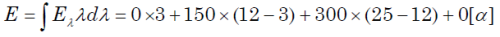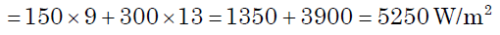QUESTION: 27

Two long parallel surfaces, each of emissivity 0.7 are maintained atdifferent temperatures and accordingly have radiation exchange between them. It is desired to reduce 75% of this radiant heat transfer by inserting thin parallel shields of equal emissivity (0.7) on both sides.What would be the number of shields?

Solution:

Ans. (c)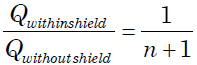or 0.25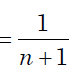or  n = 3

QUESTION: 28

Solar energy is absorbed by the wall of a building as shown in the above figure. Assuming that the ambient temperature inside and outside are equal and considering steady-state, the equivalent circuit will be as shown in (Symbols: Rco = Rconvection,outside RCI = Rconvection,inside
and Rw = RWall)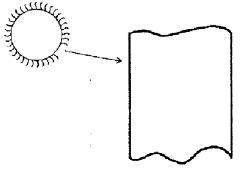Solution:

Ans. (a) All resistances are in series.

QUESTION: 29

Heat transfer by radiation between two grey bodies of emissivity ε is proportional to (notations have their usual meanings)

Solution:

Ans. (b)

QUESTION: 30

For the radiation between two infinite parallel planes of emissivity ε1 and ε2 respectively, which one of the following is the expression for emissivity factor?

Solution:

Ans. (d)